# 算法导论第七章快速排序

（1）、快速排序最坏情况下的时间复杂度为O(n^2)，虽然最坏情况下性能较差，但快排在实际应用中是最佳选择。原因在于：其平均性能较好，为O(nlgn)，且O(nlgn)记号中的常数因子较小，而且是稳定排序。

（2）、快速排序的思想和合并排序一样，即分治。快排排序的分治思想体现在：

a、首先从待排序的数中选择一个作为基数，基数的选择对于排序的性能有很大的影响，也是快排改进的关键所在

b、分治，将比基数小的数放在左边，比基数大的数放在右边。

c、对分出来的两个分区分别执行上一步，直到区间只有一个数为止。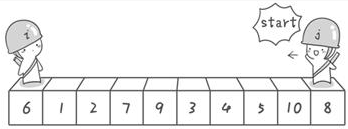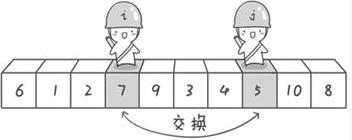1 int Hoare_Partition(int arr[], int left, int right)
2 {
3     int temp = arr[left];
4     int i = left;
5     int j = right;
6
7     while(i < j) {
8         while (arr[j] >= temp && i < j) //from right to left
9             j --;
10         while (arr[i] <= temp && i < j) //from left to right
11             i ++;
12         Swap(arr[i], arr[j]);
13     }
14     Swap(arr[left], arr[i]);
15     return i;
16 }
17
18 void Hoare_QuickSort(int arr[], int left, int right)
19 {
20     if (left < right) {
21         int mid = Hoare_Partition(arr, left, right);
22         Hoare_QuickSort(arr, left, mid-1);
23         Hoare_QuickSort(arr, mid+1, right);
24     }
25 }

 1 int Partition(int arr[], int left, int right)
2 {
3     int temp = arr[right];
4     int i = left - 1;
5
6     for (int j = left; j <= right-1; j ++) {
7         if (arr[j] <= temp) {
8             i ++;
9             Swap(arr[i], arr[j]);
10         }
11     }
12     Swap(arr[right], arr[i+1]); //!!!note: can't use temp:local variable
13     return i+1;
14 }
15
16 void QuickSort(int arr[], int left, int right)
17 {
18     if (left < right) {
19         int mid = Partition(arr, left, right);
20         QuickSort(arr, left, mid-1);
21         QuickSort(arr, mid+1, right);
22     }
23 }

1、随机优化：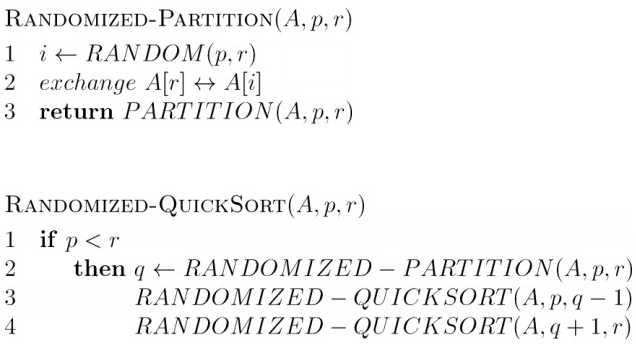2、中位数优化法：

3、递归栈的优化：

QUICKSORT算法包含两个对其自身的递归调用，即调用PARTITION后，左边的子数组和右边的子数组分别被递归排序。QUICKSORT中的第二次递归调用并不是必须的，可以用迭代控制结构来代替它，这种技术叫做“尾递归”，大多数的编译器也使用了这项技术。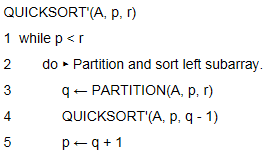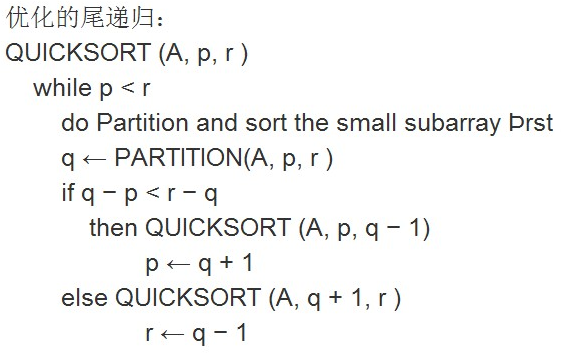1 //随机优化版本
2 //get random num between m and n;
3 int Random(int m, int n)
4 {
5     srand((unsigned int)time(0));
6     int ret = m + rand() % (n-m+1);
7     return ret;
8 }
9
10
11 void Random_QuickSort(int arr[], int left, int right)
12 {
13     int index = Random(left, right);
14
15     Swap(arr[index], arr[right]);
16     QuickSort(arr, left, right);
17 }
 1 //中位数优化，下面一个获取中位数的函数
2 //get mid num of a,b,c;
3 int MidNum(int a, int b, int c)
4 {
5     if ((a-b)*(a-c) <= 0)
6         return a;
7     else if ((b-a)*(b-c) <= 0)
8         return b;
9     else if ((c-a)*(c-b) <= 0)
10         return c;
11 }
 1 //模拟尾递归
2 void Tail_Recursive_QuickSort(int arr[], int left, int right)
3 {
4     while (left < right) { //use while not if
5         int mid = Partition(arr, left, right);
6         Tail_Recursive_QuickSort(arr, left, mid-1);
7         left = mid + 1;
8     }
9 }
10
11 //尾递归优化
12 void Tail_Recursive_QuickSort_Optimize(int arr[], int left, int right)
13 {
14     while(left < right) {
15         int mid = Partition(arr, left, right);
16         if (mid-left < right-mid) {
17             Tail_Recursive_QuickSort_Optimize(arr, left, mid-1);
18             left = mid + 1;
19         }
20         else {
21             Tail_Recursive_QuickSort_Optimize(arr, mid+1, right);
22             right = mid - 1;
23         }
24     }
25 }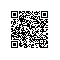使用钉钉扫一扫加入圈子
+ 订阅